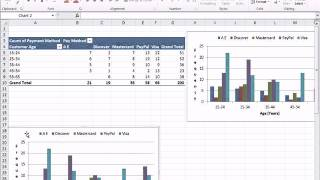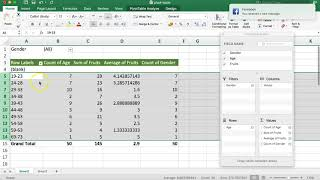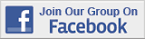Excel Tips & Tutorials:
Making Comparisons
 Suggest a Link Alphabetize Page Printer-Friendly List
Watch and Learn
Watch and Learn
Watch and Learn
Watch and Learn
 Calculating Means and Standard Deviations in PivotTableUseful for creating mean comparison tables (7:34).Cross Tabulation With Frequencies and PercentagesShows how to calculate values and format PivotTable (15:12).How to Group, Ungroup, and Remove PivotTable RowsUseful for making comparisons with interval-level independent variable (3:23).Excel Pivot Tables ExplainedLeila Gharani uses PivotTables to summarize sales data by other variables, like sales by product (13:21).Calculating Means and Standard Deviations in PivotTableUseful for creating mean comparison tables (7:34).Cross Tabulation With Frequencies and PercentagesShows how to calculate values and format PivotTable (15:12).How to Group, Ungroup, and Remove PivotTable RowsUseful for making comparisons with interval-level independent variable (3:23).Calculating Means and Standard Deviations in PivotTableUseful for creating mean comparison tables (7:34).Cross Tabulation With Frequencies and PercentagesShows how to calculate values and format PivotTable (15:12).Calculating Means and Standard Deviations in PivotTableUseful for creating mean comparison tables (7:34).Over 18,000 Members!